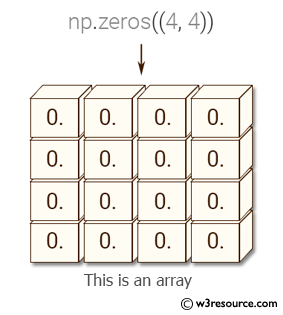﻿ NumPy: Print all the values of an array - w3resource# NumPy: Print all the values of an array

## NumPy: Array Object Exercise-97 with Solution

Write a NumPy program to print all the values of an array.

Pictorial Presentation:Sample Solution:

Python Code:

``````import numpy as np
np.set_printoptions(threshold=np.nan)
x = np.zeros((4, 4))
print(x)
```
```

Sample Output:

```[[ 0.  0.  0.  0.]
[ 0.  0.  0.  0.]
[ 0.  0.  0.  0.]
[ 0.  0.  0.  0.]]
```

Python Code Editor:

Have another way to solve this solution? Contribute your code (and comments) through Disqus.

What is the difficulty level of this exercise?

Test your Python skills with w3resource's quiz

﻿

## Python: Tips of the Day

Set comprehension:

```>>> m = {x ** 2 for x in range(5)}
>>> m
{0, 1, 4, 9, 16}
```